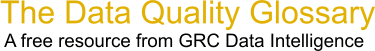Choose Index below for a list of all words and phrases defined in this glossary.

# Coefficient of Variation

index | Index

## Coefficient of Variation - definitions

Coefficient of variation - The coefficient of variation is is defined by the ratio of the standard deviation to the mean. The coefficient of variation is important because it adds context to a standard deviation by comparing it to the mean. For example, look at the following observations:

```   A) Standard Deviation = 10, Mean = 100
B) Standard Deviation = 10, Mean = 1,000
```

Observation A has a much greater spread around the mean than observation B.

Source: NextMark, 23 July 2009 13:04:25, https://www.nextmark.com/resources/glossary-of-terms/ ?glossaryTermId=a0800000000FVTYAA4These advertisers support this free service

Coefficient of variation - Coefficient of variation is defined as the relative measure of dispersion it relates the mean and standard deviation by expressing the Std deviation as a % of mean. The benefit of standard deviation is a absolute measure which explains the dispersion in the same unit as original data.

[Category=Data Quality ]

Source: iSixSigma, 07 January 2011 08:57:46, http://www.isixsigma.com/index.php?option=com_glossaryData Quality Glossary.  A free resource from GRC Data Intelligence. For comments, questions or feedback: dqglossary@grcdi.nl